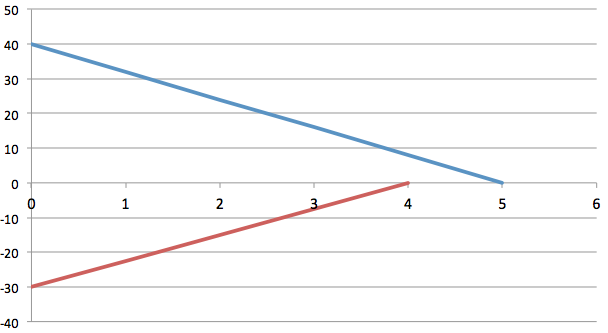# Distance between two trainsTwo trains move along a track with their respective velocities $+40$ m/s and $-30$ m/s. Suddenly, the conductors notice that they are headed toward each other. The above graph gives each train's velocity $v$ as a function of time $t,$ as the conductors slow down their trains. The slowing processes begin when the two trains are $200$ m apart. What is the distance (in m) between the two trains when both trains have stopped?

The graph's vertical scaling is set by $v_{s}= 40.0$ m/s.

×

Problem Loading...

Note Loading...

Set Loading...